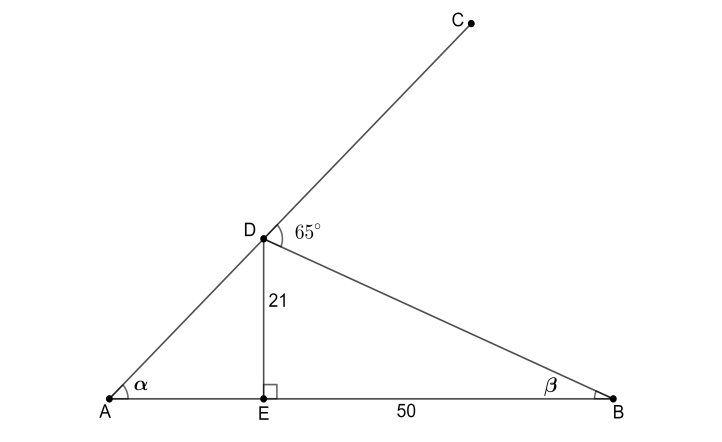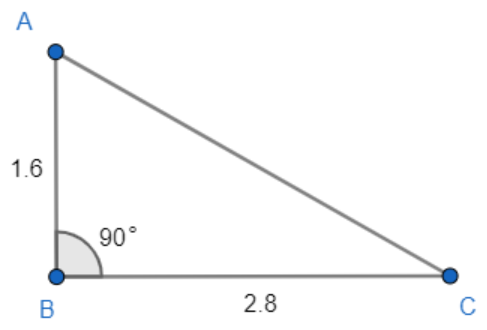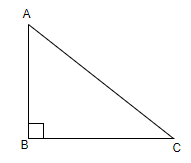Filters
Latest Questions
Mathematics
Concept of perpendicular base and hypotenuse in a right triangles
Find the angle $\alpha$ .Mathematics
Concept of perpendicular base and hypotenuse in a right triangles
If the length of shadow of a pole is equal to the height of the pole, then the angle of elevation of the sun is: -
(a) ${{30}^{\circ }}$
(b) ${{75}^{\circ }}$
(c) ${{60}^{\circ }}$
(d) ${{45}^{\circ }}$
Mathematics
Concept of perpendicular base and hypotenuse in a right triangles
ABC is a right angled triangle , $\left| \!{\underline {\, C \,}} \right. = {90^ \circ }$. If P is the perpendicular from C to AB and a,b,c have the usual meaning , then prove that $\dfrac{1}{{{p^2}}} = \dfrac{1}{{{a^2}}} + \dfrac{1}{{{b^2}}}$.
Mathematics
Concept of perpendicular base and hypotenuse in a right triangles
In triangle $ABC$ angle $B = {90^ \circ }$and $BC = 5\;{\text{cm}},\;AC - AB = 1$. Evaluate $\dfrac{{1 + \sin C}}{{1 + \cos C}}$.

Mathematics
Concept of perpendicular base and hypotenuse in a right triangles
In triangle ABC, right-angled at B, if $tan{\rm{A}} = \dfrac{1}{{\sqrt 3 }}$, find the value of:
i) $\sin {\rm{A}}\cos {\rm{C}} + \cos {\rm{A}}\sin {\rm{C}}$
ii) $cos{\rm{A}}\cos {\rm{C}} - \sin {\rm{A}}\sin {\rm{C}}$

Mathematics
Concept of perpendicular base and hypotenuse in a right triangles
If $3cot{\rm{A}} = 4$, check whether $\dfrac{{1 - {{\tan }^2}{\rm{A}}}}{{1 + {{\tan }^2}{\rm{A}}}} = {\cos ^2}{\rm{A}} - {\sin ^2}{\rm{A}}$ or not.

Mathematics
Concept of perpendicular base and hypotenuse in a right triangles
Calculate the size of $\angle BAC$ for the following image.Mathematics
Concept of perpendicular base and hypotenuse in a right triangles
In a right-angled triangle $\Delta ABC$, the lengths of two sides are 8 cm and 6 cm, then which among the given statements is/are correct?(a) Length of greatest side is 10 cm
(b) Angle ACB is greater than ${{90}^{\circ }}$
(c) Angle BAC is less than ${{90}^{\circ }}$
(d) Pythagoras theorem is not applicable here.
Mathematics
Concept of perpendicular base and hypotenuse in a right triangles
Which of the following is not defined?
A. $\sin 90{}^\circ$
B. $\tan 0{}^\circ$
C. $\cot 90{}^\circ$
D. $\text{cosec }0{}^\circ$

Mathematics
Concept of perpendicular base and hypotenuse in a right triangles
ABC and ADC are the two right triangles with common hypotenuse AC. Prove that $\angle CAD = \angle CBD$.
Mathematics
Concept of perpendicular base and hypotenuse in a right triangles
The hypotenuse of a right triangle is 25 cm. The difference between the lengths of the other two sides of the triangle is 5 cm. Find the perimeter of the triangle.
Mathematics
Concept of perpendicular base and hypotenuse in a right triangles
The angle of elevation of a ladder leaning against a wall is ${{60}^{\circ }}$and the foot of the ladder is 4.6 meters away from the wall. The length of the ladder is:
(a) 2.3 meters
(b) 4.6 meters
(c) 7.8 meters
(d) 9.2 meters
Prev
1
2